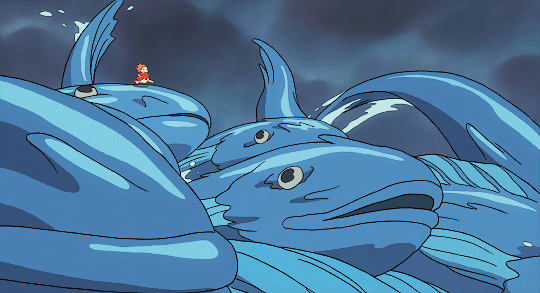?二值化处理

?膨胀、腐蚀

?开运算、闭运算

Step1：灰度处理

Step2：对视频进行帧差处理

Step3：二值化处理

Step4：腐蚀处理

Step5：膨胀处理

Step6：标记、框选目标

?完整代码

# 一、图像处理## ?二值化处理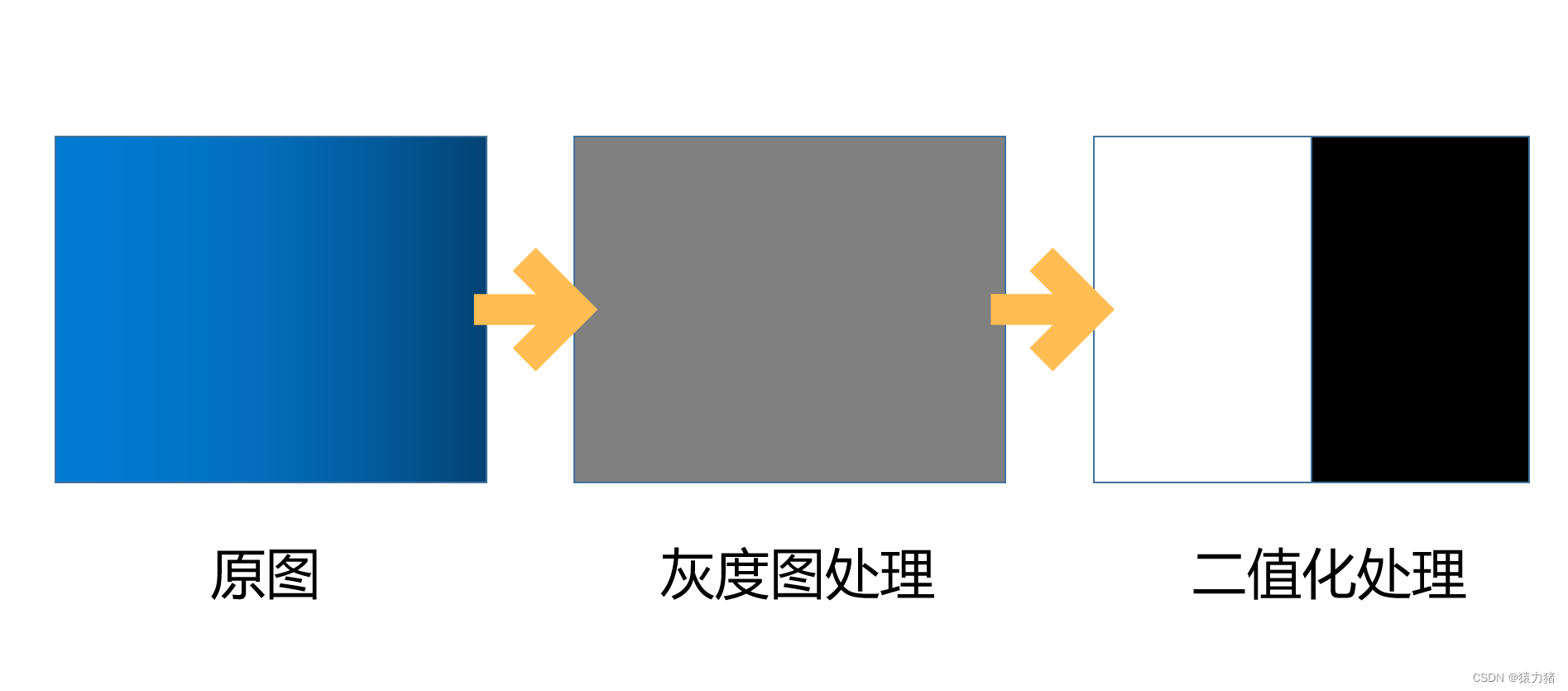?作用：

• 图像的二值化就是将图像上的像素点的灰度值设置为0或255，这样将使整个图像呈现出明显的黑白效果
• 在数字图像处理中，二值图像占有非常重要的地位，图像的二值化使图像中数据量大为减少，从而能凸显出目标的轮廓

?全局阈值：

?局部阈值：

（即二进制阈值或反二进制阈值）

## ?膨胀、腐蚀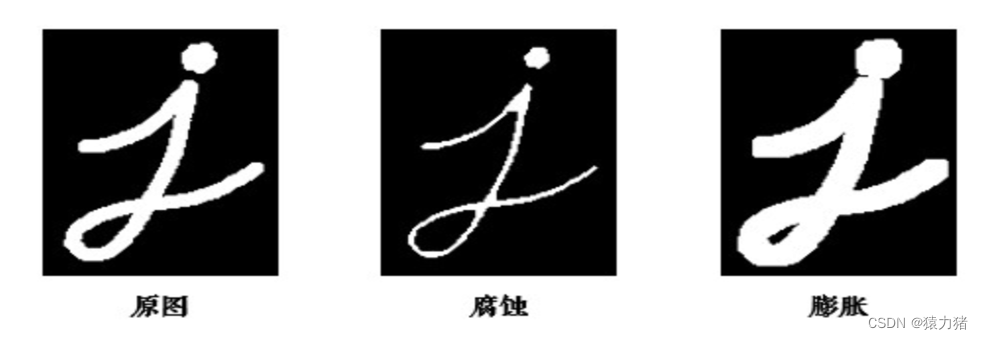• 膨胀和腐蚀是针对图片的二值化数据进行操作的主要是针对高亮部分
• 膨胀就是使用算法，将图像的边缘扩大些，作用就是将目标的边缘或者是内部的坑填掉
• 腐蚀就是使用算法，将图像的边缘腐蚀掉，作用就是将目标的边缘的“毛刺”踢除掉

1️⃣膨胀过程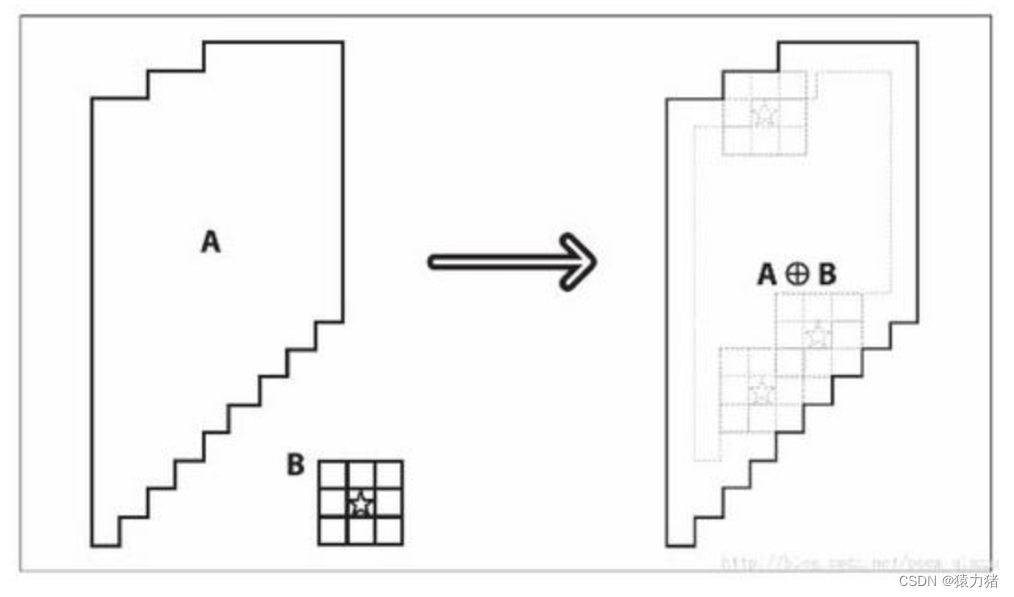2️⃣腐蚀过程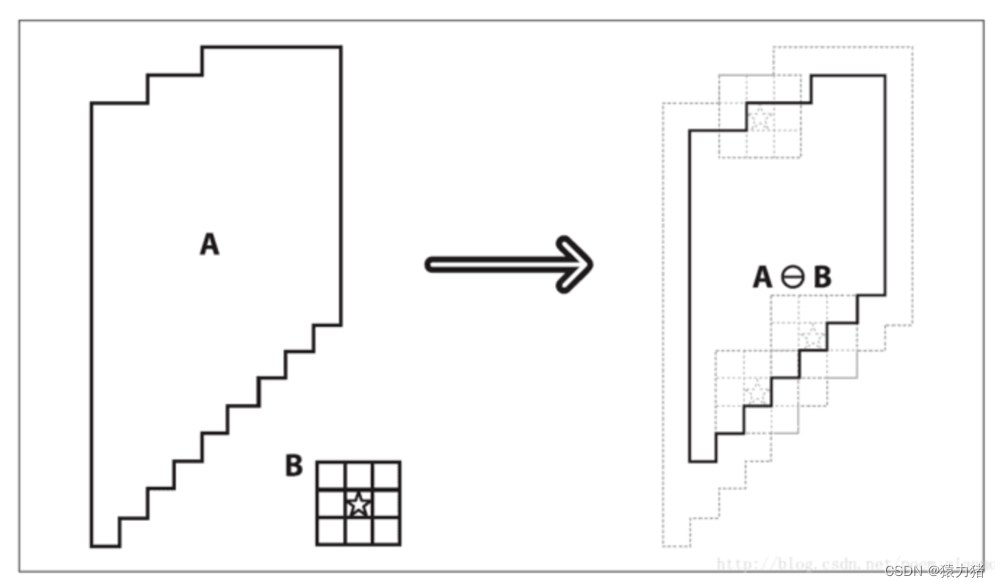## ?开运算、闭运算

1️⃣开运算：先腐蚀再膨胀，用来消除小物体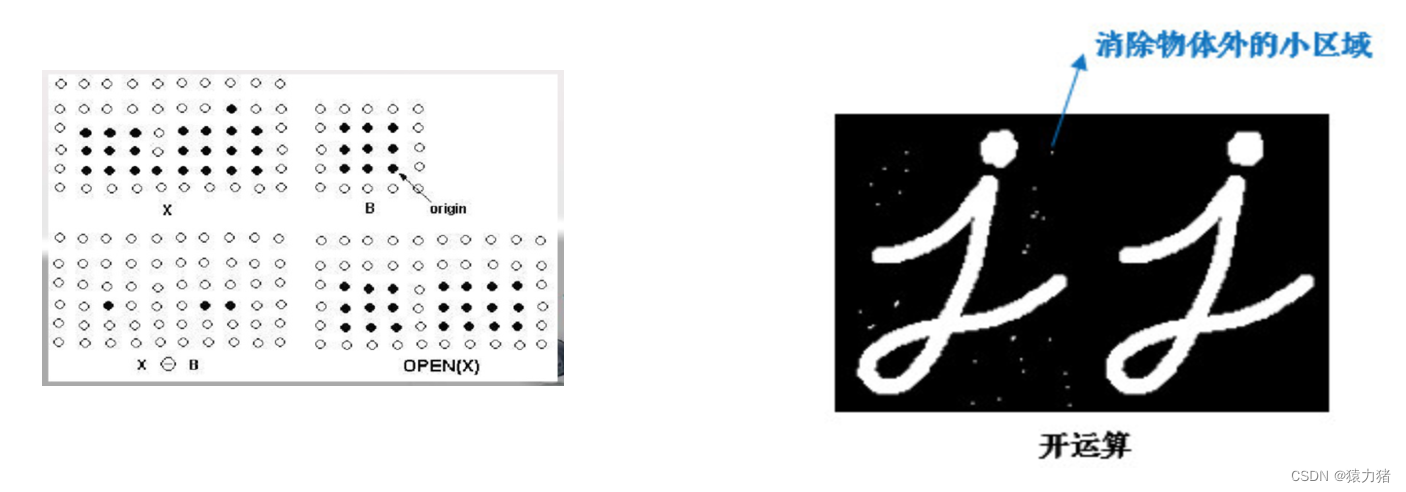2️⃣闭运算： 先膨胀再腐蚀，用于排除小型黑洞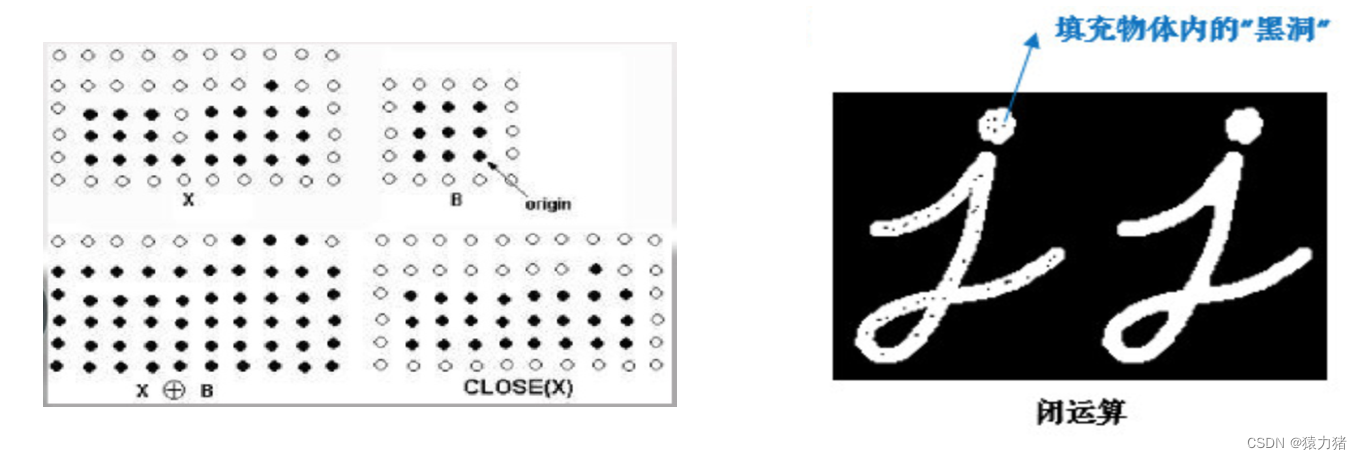# 二、案例实现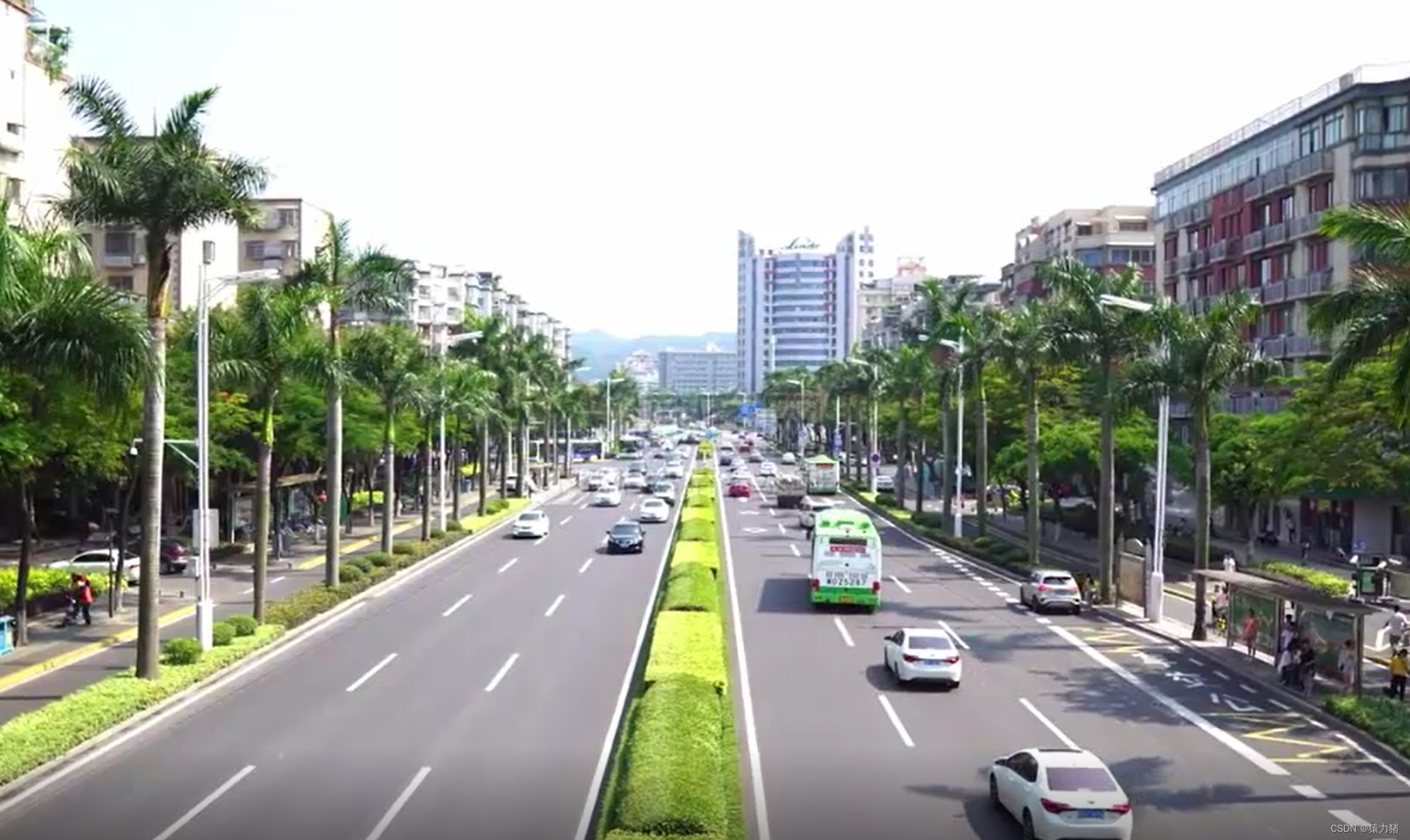## Step1：灰度处理

• 将传入的两帧先进行处理，先将其转化成RGB类型图片再转化为灰度图
`` cvtColor(frontFrame,frontGray,CV_BGR2GRAY);//前一帧灰度处理 cvtColor(afterFrame,afterGray,CV_BGR2GRAY);//后一帧灰度处理``

## Step2：对视频进行帧差处理

• 将两帧图片进行帧差处理，将对比结果存储在diff中
`` //【帧差法】对比两帧图像之间差异，捕获运动物体 //缺点:所有运动的物体都会展现 Mat diff; absdiff(frontGray,afterGray,diff);//前后两帧对比存在diff中``
• 灰度图 + 帧差处理后，效果如下：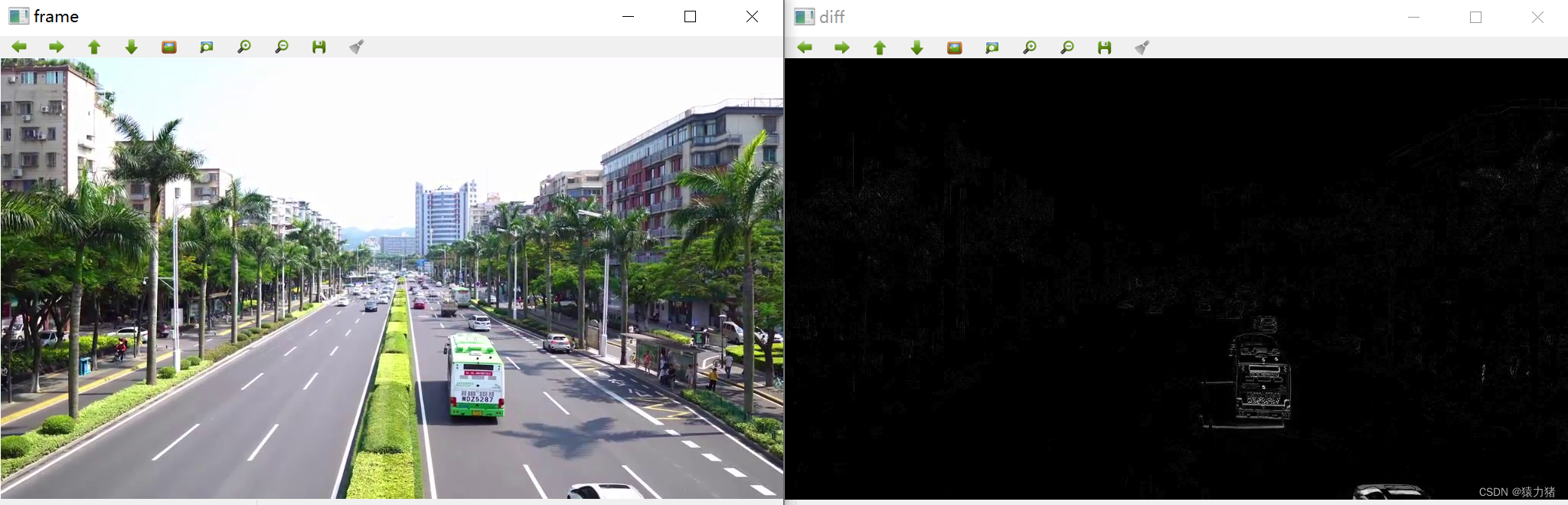## Step3：二值化处理

• 我们可以看到，公交车的识别区块并不是很明显，因此，接下来我们对视频进行二值化处理
`` //【二值化】黑白分明 //局部阈值 threshold(diff,diff,25,255,CV_THRESH_BINARY);``
• 黑白更加分明，效果如下：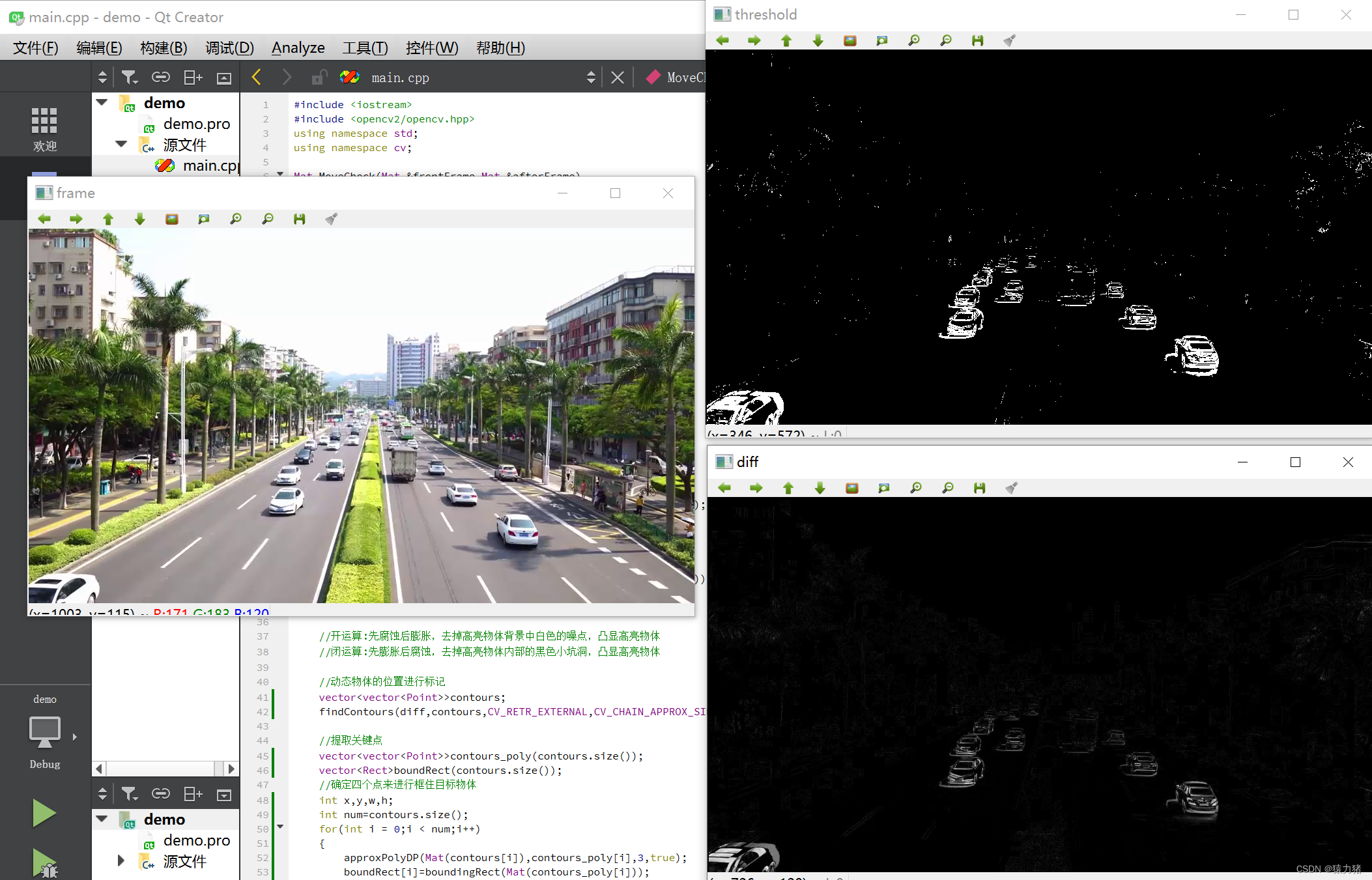## Step4：腐蚀处理

• 我们会发现，二值化处理后，比原来多出好多的白色的小点，这时候我们就需要进行腐蚀处理，腐蚀掉<x*x方块大小的像素，这里设置了4*4，来清除大部分噪点
`` //【腐蚀处理】将背景中的白色噪点尽可能去除 降噪处理 Mat element = cv::getStructuringElement(MORPH_RECT,Size(4,4));//小于4*4方块的白色噪点都会被腐蚀 erode(diff,diff,element);``
• 腐蚀处理之后，效果如下：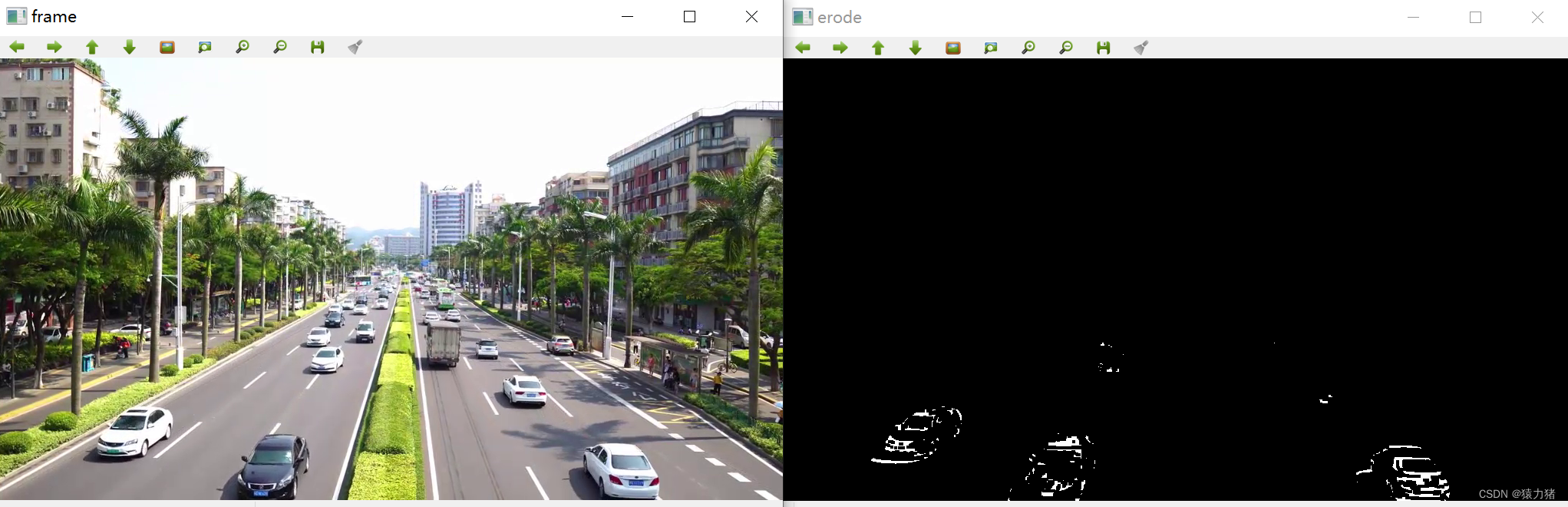## Step5：膨胀处理

• 噪点相较于之前确实少了很多，但是运动的物体也被腐蚀掉了很多，可能会识别不到一些移动车辆，这里我们继续进行膨胀处理，将我们的目标像素图像变“胖”
`` //【膨胀处理】将白色区域扩大,更加明显，利于目标识别 Mat element2=cv::getStructuringElement(MORPH_RECT,Size(30,30)); dilate(diff,diff,element2);``
• 膨胀处理后，效果如下：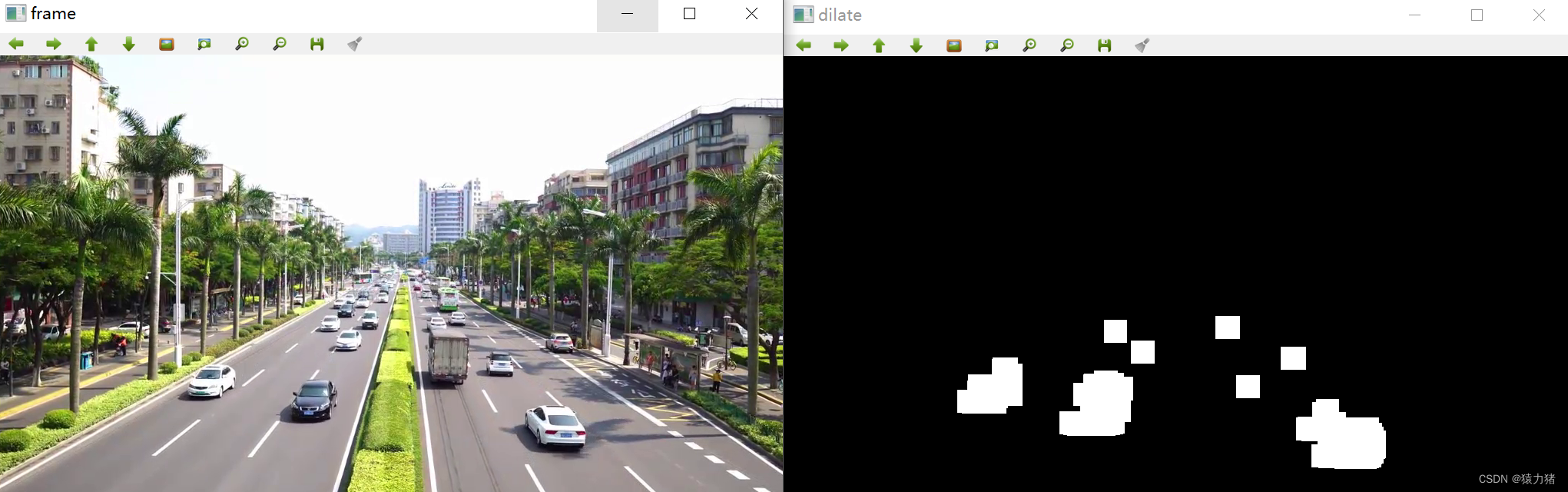## Step6：标记、框选目标

• 最后，将我们的目标像素图像进行标记，并且加一个框把移动的物体框选出来
`` //动态物体的位置进行标记 vector<vector<Point>>contours; findContours(diff,contours,CV_RETR_EXTERNAL,CV_CHAIN_APPROX_SIMPLE,Point(0,0)); //提取关键点 vector<vector<Point>>contours_poly(contours.size()); vector<Rect>boundRect(contours.size()); //确定四个点来进行框住目标物体 int x,y,w,h; int num=contours.size(); for(int i = 0;i < num;i++) { approxPolyDP(Mat(contours[i]),contours_poly[i],3,true); boundRect[i]=boundingRect(Mat(contours_poly[i])); x=boundRect[i].x; y=boundRect[i].y; w=boundRect[i].width; h=boundRect[i].height; //绘制矩形 rectangle(resFrame,Point(x,y),Point(x+w,y+h),Scalar(255,0,0),2);//Scalar颜色 }``
• 完成上述所有步骤后，效果如下：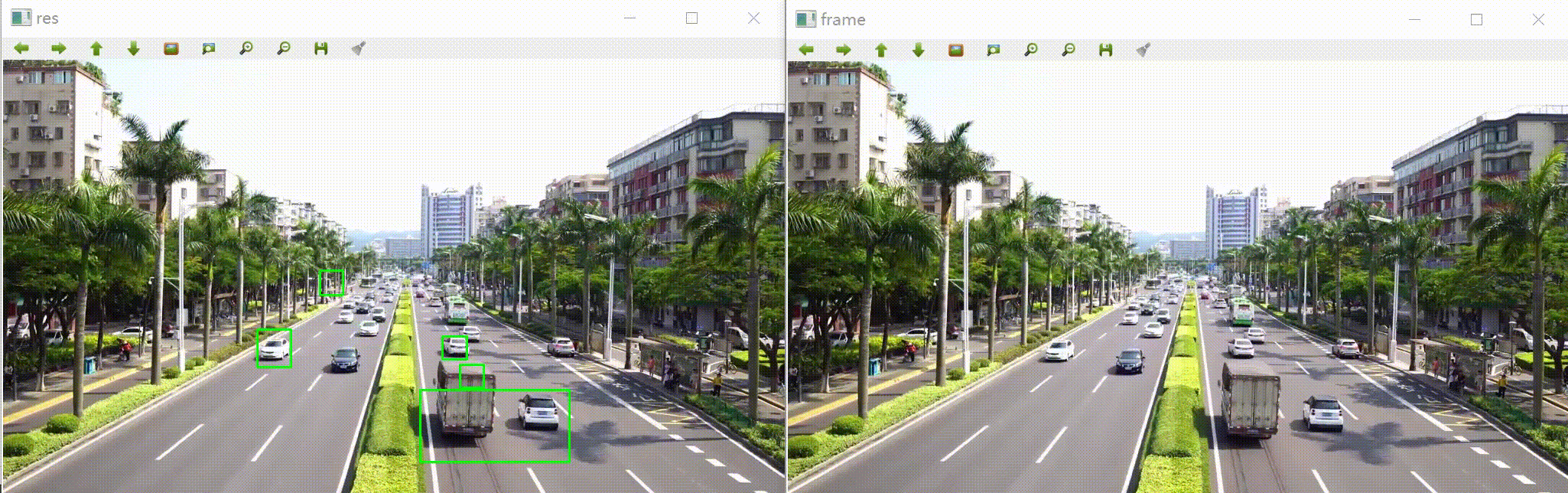## ?完整代码

``#include <iostream>#include <opencv2/opencv.hpp>using namespace std;using namespace cv;Mat MoveCheck(Mat &frontFrame,Mat &afterFrame){ Mat frontGray,afterGray;//前后灰度处理 Mat resFrame = afterFrame.clone(); //【灰度处理】 cvtColor(frontFrame,frontGray,CV_BGR2GRAY);//前一帧灰度处理 cvtColor(afterFrame,afterGray,CV_BGR2GRAY);//后一帧灰度处理 //【帧差法】对比两帧图像之间差异，捕获运动物体 //缺点:所有运动的物体都会展现 Mat diff; absdiff(frontGray,afterGray,diff);//前后两帧对比存在diff中 //imshow("diff",diff); //【二值化】黑白分明 //局部阈值 threshold(diff,diff,25,255,CV_THRESH_BINARY); //imshow("threshold",diff); //【腐蚀处理】将背景中的白色噪点尽可能去除 降噪处理 Mat element = cv::getStructuringElement(MORPH_RECT,Size(4,4));//小于4*4方块的白色噪点都会被腐蚀 erode(diff,diff,element); //imshow("erode",diff); //【膨胀处理】将白色区域扩大,更加明显，利于目标识别 Mat element2=cv::getStructuringElement(MORPH_RECT,Size(30,30)); dilate(diff,diff,element2); //imshow("dilate",diff); //开运算:先腐蚀后膨胀，去掉高亮物体背景中白色的噪点，凸显高亮物体 //闭运算:先膨胀后腐蚀，去掉高亮物体内部的黑色小坑洞，凸显高亮物体 //动态物体的位置进行标记 vector<vector<Point>>contours; findContours(diff,contours,CV_RETR_EXTERNAL,CV_CHAIN_APPROX_SIMPLE,Point(0,0)); //提取关键点 vector<vector<Point>>contours_poly(contours.size()); vector<Rect>boundRect(contours.size()); //确定四个点来进行框住目标物体 int x,y,w,h; int num=contours.size(); for(int i = 0;i < num;i++) { approxPolyDP(Mat(contours[i]),contours_poly[i],3,true); boundRect[i]=boundingRect(Mat(contours_poly[i])); x=boundRect[i].x; y=boundRect[i].y; w=boundRect[i].width; h=boundRect[i].height; //绘制矩形 rectangle(resFrame,Point(x,y),Point(x+w,y+h),Scalar(255,0,0),2);//Scalar颜色 } return resFrame;}int main(int argc, char *argv[]){ Mat frame; Mat temp; Mat res; int count = 0; VideoCapture cap("C:/Users/86177/Desktop/image/carMove.mp4");//视频路径 while(cap.read(frame)) { count++; if(count == 1) { res = MoveCheck(frame,frame); } else { res = MoveCheck(temp,frame); } temp = frame.clone(); imshow("frame",frame); imshow("res",res); waitKey(25);//延时 } return 0;}``

# 三、总结

• 本文主要讲解了OpenCV图像处理基本的原理，包括帧差法二值化膨胀腐蚀开运算闭运算
• 以车辆识别为例子，详细讲解了图像处理相关操作，在各个步骤是怎么样的一个效果
• 车辆识别在我们日常生活中非常常见，是一个很经典的案例，本案例算是初步实现，但是，通过案例也发现，帧差法的缺点显而易见，测试视频存在很多干扰，比如说其他移动的物体，例如，风比较大，树叶跟着动，我们的框选位置就会受到干扰，不一定是我们预期想要的目标，接下来也会继续对这方面进行深入探究和学习，争取做到更好！